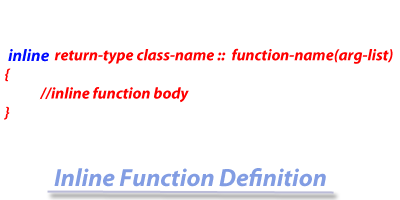< Prev
Next >

# C++ Inline Function

An inline function is a feature of C++ which rules out the overheads associated with the calls to small functions. In order to understand why we use inline functions and the overheads associated with a function call, let's see a normal function call scenario.

Suppose we have to call a function Y from within the currently executing function X. In such a case, there are a few of overheads associated with the function call, such as - jumping from function X to function Y, copying the arguments of function Y into the stack, returning back to function X(once function Y has finished its execution) and more.

Hence, to overcome these overheads(which requires a lot of time and memory) associated with the calling of a normal function, C++ provides us the feature of using an inline function.

## How an inline function is different from a normal function?

An inline function is a function that contains a few lines of statements, which are expanded in the same line in which an inline function is called or invoked. Hence, the overhead associated with the calling of a normal function such as - jumping to the calling function and return is ruled out when an inline function is called.

Note : As the call to an inline function is replaced by its definition, hence an inline function definition should not be contain more than a single or two statements otherwise its significance will be lost.

## Syntax of an inline function

In order to define an inline function, we have to prefix a function header(when a function is defined) with an inline keyword. Let us see the syntax -• The return-type is the return-type of an inline function.
• The class-name is the name of the class which an inline function is part of.
• The :: is the scope resolution operator.
• The function-name is the name of the inline function.
• The arg-list is name and type of arguments allowed to be passed to this function.

## Example of inline function

``````//An inline function

#include<iostream>

using namespace std;

class A
{
public:
int a = 10;

void add(int); // function prototype declaration

};

// function defintion
inline void A :: add(int x)
{
a = a + x;
}

int main()
{
A ob;
cout<< "The value in a is : " << ob.a << "\n";

cout<< "After the add function is called " << "\n";
cout<< "The value in a is : " << ob.a << "\n";

return 0;
}``````

## Output-

``````The value in a is : 10
After the add function is called
The value in a is : 20``````

## Program Analysis

Call to add() inline function, such as -

``add(10);``

is replaced by the function definition of add() inline function i.e. a single statement which comprises its definition, in the same line in which the inline function was called.

``a = a + x; ``

## Multiple inline functions

We could create any number of inline functions in a program.

``````//An inline function

#include

using namespace std;

class A
{
public:
int a = 10;
float b = 5.5f;

void display_a(); // function prototype declaration
void display_b(); // function prototype declaration

};

// inline function defintion
inline void A :: display_a()
{
cout<<"The value of a is : " << a << "\n";
}

// inline function defintion
inline void A :: display_b()
{
cout<<"The value of b is : " << b << "\n";
}

int main()
{
A ob;
ob.display_a();
ob.display_b();
return 0;
}``````

## Output-

``````The value of a is : 10
The value of b is : 5.5``````

## Program Analysis

Call to display_a() inline function, such as -

• ``display_a();``

• is replaced by the function definition of display_a() inline function i.e. a single statement which comprises its definition, in the same line in which the inline function was called.

``cout<<"The value of a is : " << a << "\n"; ``

Call to display_b() inline function, such as -

• ``display_b();``

• is replaced by the function definition of display_a() inline function i.e. a single statement which comprises its definition, in the same line in which the inline function was called.

``cout<<"The value of b is : " << b << "\n"; ``